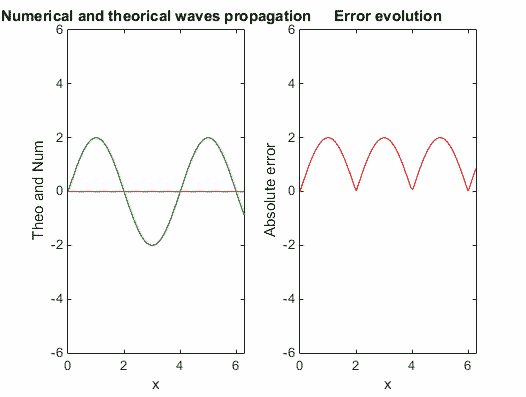# Vibratring string with forced excitation.

    clear all; clf


## Initialisation of parameters

    % parameters
N=150; % number of gridpoints
L=2*pi; % domain length
c=1; % wave velocity
dt=0.1; % time step
tmax=4*pi; % final time

% differentiation matrices
scale=-2/L;
[x,DM] = chebdif(N,2);
dx=DM(:,:,1)*scale;
dxx=DM(:,:,2)*scale^2;
x=(x-1)/scale;
Z=zeros(N,N); I=eye(N);
II=eye(2*N);

% system matrices
E=[I,Z; Z,I];
A=[Z,I; c^2*dxx,Z];
C=[Z(1,:),dx(1,:); Z(N,:), dx(N,:)];

% locations in the state
v=1:N;
f=N+1:2*N;

## Boundary conditions

Just like in the original code, the system has one variable and two time derivatives, so we should apply two boundary conditions. We will simply say that the position of the string at its both ends should be static: two attachment points. This part only consists in applying homogeneous Dirichlet conditions on the first and last point of the state vector for the state position. Later (in the march in time), we will change the state of the first point.

    loc=[f(1),f(N)];

E(loc,:)=0;

A(loc,:)=II(loc,:);

% initial condition
q=[zeros(N,1);zeros(N,1)];


## March in time matrix

    M=(E-A*dt/2)\(E+A*dt/2);

% marching loop
tvec=1:dt:tmax+1;
Nt=length(tvec);
e=zeros(Nt,1);
b=0*q;

## Resolution

To resolve the forced vibrated string system, we have to add a vector b, which will simulate an excitation on the first point. Consequently, the resolution follows this form :

    for ind=1:Nt

t=dt*(ind-1);
b(loc(1))=2*sin((1/2*pi)*t);
H=E-A*dt;
G=b*dt;
q = M*q + H\G ;
e(ind)=interp1(x,q(f),L/2); % store center point


Here we create the established theoretical solution. Before: t=2\pi the wave travels on the string. (like an advection of a quantity) The theoretical solution is then f(x,t)= -2*sin((1/2*pi)*(c*t-x)) At : t=2\pi the wave is reflected. The established theoretical solution should now contain the superposition of the two wave. The one coming from the first point and the reflected one.

    ftheo((c*t-x)<0)=0;
if t<2*pi
ftheo=-2*sin((1/2*pi)*(c*t-x));
else
ftheo=2*sin((1/2*pi)*(c*t+x-4*pi))-2*sin((1/2*pi)*(c*t-x));
end


Plotting
We have recorded the wave propagation and the evolution of the absolute difference between the numerical and theoretical solutions.

    figure (1)
subplot(1,2,1);
plot(x,q(f),'b',x,q(v),'r--',x,ftheo,'g')
axis([0,L,-6,6])
title('Numerical and theorical waves propagation')
xlabel('x')
ylabel('theo and q')
set(gcf,'color','w'); % set figure background to white
drawnow;

subplot(1,2,2)
plot(x,abs(q(f)-ftheo),'r')
title('error evolution');
axis([0,L,-6,6])
xlabel('x');
ylabel('absolute error')
drawnow;


GIF creation code

    %     frame = getframe(1);
%     im = frame2im(frame);
%     [imind,cm] = rgb2ind(im,256);
%     outfile = 'vibratring_string_forced.gif';
%
%     % On the first loop, create the file. In subsequent loops, append.
%     if ind==1
%         imwrite(imind,cm,outfile,'gif','DelayTime',0,'loopcount',inf);
%     else
%         imwrite(imind,cm,outfile,'gif','DelayTime',0,'writemode','append');
%
%
%     end

% End of the resolution
end


With this plotting, we can visualize the amplitude evoluton of waves superposition.

## Observations

We can observe that after a certain number of reflection ( around 14 “goings and comings”), the different waves created by the excitation and reflected ones bring into complete opposition. The string is then standing totally flat for a short moment, while the velocity is non-existant.

This phenomenon can be explained by the fact that the simulated system is not dissipative.While the string is excited by a frequency which put the waves on opposite phase.

## Figures%}